Comprehensive measurement of pp-chain solar neutrinos
Nature volume 562pages 505–510 (2018)
Published:
[doi:10.1038/s41586-018-0624-y] (full text here)

## Data availability

The datasets generated during the current study are freely available: see below (scroll down). Additional information is available from the Borexino Collaboration spokesperson (spokesperson-borex@lngs.infn.it) upon reasonable request.

## News Coverage:

INFN Press release:

English: “Over 10 years of scientific successes: thanks to Borexino today we know the Sun with unprecedented detail
Italian: “Oltre 10 anni di successi scientifici: grazie a Borexino oggi conosciamo il Sole con un dettaglio senza precedenti

A first ‘snapshot’ of the complete spectrum of neutrinos emitted by the sun
Neutrinos sunny side up
Solar neutrinos reveal how the Sun shines

## Abstract

About 99 per cent of solar energy is produced through sequences of nuclear reactions that convert hydrogen into helium, starting from the fusion of two protons (the pp chain). The neutrinos emitted by five of these reactions represent a unique probe of the Sun’s internal working and, at the same time, offer an intense natural neutrino beam for fundamental physics. Here we report a complete study of the pp chain. We measure the neutrino–electron elastic-scattering rates for neutrinos produced by four reactions of the chain: the initial proton–proton fusion, the electron-capture decay of beryllium-7, the three-body proton–electron–proton (pep) fusion, here measured with the highest precision so far achieved, and the boron-8 beta decay, measured with the lowest energy threshold. We also set a limit on the neutrino flux produced by the 3He–proton fusion (hep). These measurements provide a direct determination of the relative intensity of the two primary terminations of the pp chain (pp-I and pp-II) and an indication that the temperature profile in the Sun is more compatible with solar models that assume high surface metallicity. We also determine the survival probability of solar electron neutrinos at different energies, thus probing simultaneously and with high precision the neutrino flavour-conversion paradigm, both in vacuum and in matter-dominated regimes.

## Open Data

In autumn 2018, the Borexino collaboration publishes [Nature 562, 505 (2018)] the first complete study of all neutrinos components  from the proton-proton fusion process in the Sun.

About 99% of the solar energy is produced through sequences of nuclear reactions, initiated by the so-called pp (proton-proton) fusion, in which hydrogen is converted into helium. Neutrinos emitted by this nuclear fusion chain represent a unique tool for solar and neutrino physics.

Here we report the first comprehensive measurement of the interaction rates of pp,Be, and pep neutrinos with the highest precision to date, together with that ofB neutrinos with the lowest-threshold. We also set a limit on the hep neutrino flux.

These measurements provide  a first indication that the temperature profile in the Sun is more compatible with solar models assuming high surface metallicity, and a direct determination of the pp-II/pp-I  branching ratio. At the same time, we determined the survival probability of solar electron neutrinos at different energies, thus probing simultaneously and with high precision the MSW-LMA flavor conversion paradigm in both the vacuum and the matter dominated regimes.This plot (as given in Fig. 2a of the 2018 Nature paper) reports the distribution of events after selection cuts, and the fit of the Borexino Low-Energy-Region (LER, [0.19-2.93] MeV) spectrum. Neutrino and background components are depicted over the Three Fold Coincidence (TFC) subtracted energy spectrum with suppressedC background. The properties of the data bins and residuals are available here. For this specific fit we also report the correlation matrix for both the Monte Carlo and the analytical approach.As regards the High-Energy-Region (HER, [3.2-16] MeV) of the Borexino spectrum, this plot (Fig. 2b of the 2018 Nature paper) reports the radial distribution of events belonging to HER-I ([3.2-5.7] MeV), after selection cuts. Fit with neutrino and background components are depicted as well. Data bins content and residuals are available here

Borexino provides the most precise measurement of the electron neutrino survival probability (P) in the low energy region, and it is so far the only experiment being able to simultaneously test neutrino flavour conversion in both the vacuum and the matter dominated regime.As in Fig. 3 of  Nature (2018), the above plot shows the  electron neutrino survival probability as a function of neutrino energy. We report the Pfrom the Borexino experimental measurements of pp,Be, pep andB solar neutrino flux, as well as the ± 1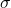theoretical prediction of Paccording to the MSW-LMA and Vacuum-LMA solutions. The theoretical bands contours are reported here while Borexino experimental Ppoints can be found here.

To further probe the robustness of the Borexino results,  we performed a statistical study to compare our Pmeasurements with two theoretical oscillation scenarios: the MSW-LMA and the Vacuum-LMA.  The test was carried out as a frequentist analysis where we adopted a test statistics t based on the ratio between the likelihood obtained assuming the MSW-LMA and the Vacuum-LMA oscillation hypothesis. This ratio test pointed out how our data disfavour the Vacuum-LMA hypothesis at 98.2% C.L., and are in excellent agreement with the expectation from the MSW-LMA paradigm.The plot above as in “Extended Data” Fig. 2 of our 2018 Nature paper (online version only)  shows the probability distribution of the test statistics t, obtained by simulating thousands of sets of Pvalues (at the pp,Be, pep andB energies) in the MSW-LMA and the Vacuum-LMA hypothesis. Data bins content for the two distributions can be found here. The dotted black line corresponds to the actual Borexino  Presults, which gives a value of=-4.16 .This plot (Fig. 4 of the Borexino 2018 Nature paper) compares the Borexino experimental results with the theoretical predictions from the low metallicity (SSM-LZ) and high metallicity (SSM-HZ) Standard Solar Models in the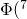Be)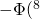B) space. Allowed contour obtained by combining the Borexino new results with all solar and KamLAND data, and leaving free the oscillation parameters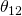and, is also shown.  All contours correspond to 68.27% C.L.
Best fit values are available here while the global analysisprofile can be found here. The contours describing the Borexino measurements and the SSM-HZ (SSM-LZ) expectations are obtained taking into account the specificBe) and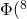B) evaluations (the best fit values and the related uncertainties) as well as their correlation, if any.

The Borexino results on theBe andB solar neutrino fluxes are compatible with the temperature profiles predicted by both the  high and low metallicity Standard Solar Models. However, the combination of the Borexino measurements provides an interesting hint in favour of the solar temperature profile predicted by the SSM-HZ. This results was obtained by performing both a frequentist and a Bayesian hypothesis test. As regards the frequentist analysis, our data disfavour the SSM-LZ at 96.6% C.L., a constraint which is slightly stronger than our sensitivity (median sensitivity, 94.2% C.L.). The Bayesan analysis confirms the mild preference for the SSM-HZ, yielding a Bayes factor of 4.9.The above plot, as in “Extended Data” Fig. 3 of the 2018 Nature paper (online version only),  shows the probability distribution of the frequency test for the SSM-LZ and the SSM-HZ. The test statistics t is obtained by simulating thousands of fake sets ofBe–B values in the HZ and LZ hypothesis. Data bins content for the two distributions can be found here. The dotted black line corresponds to the actual BorexinoBe andB results, which gives a value of=-3.49 .

Comprehensive measurement of pp-chain solar neutrinos